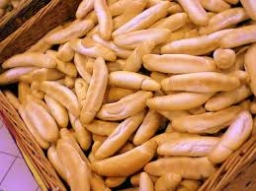# The bun

The bun costs CZK 2, and the rolls CZK 3.5. Hanka bought 22 pieces of bread, bought only buns and rolls, and paid CZK 65. how many rolls did she buy, and how many rolls?

h =  8
r =  14

### Step-by-step explanation:

2h+3.5r = 65
h+r=22

2·h+3.5·r = 65
h+r=22

2h+3.5r = 65
h+r = 22

Row 2 - 1/2 · Row 1 → Row 2
2h+3.5r = 65
-0.75r = -10.5

r = -10.5/-0.75 = 14
h = 65-3.5r/2 = 65-3.5 · 14/2 = 8

h = 8
r = 14

Our linear equations calculator calculates it.Did you find an error or inaccuracy? Feel free to write us. Thank you!

Tips for related online calculators
Do you have a system of equations and looking for calculator system of linear equations?